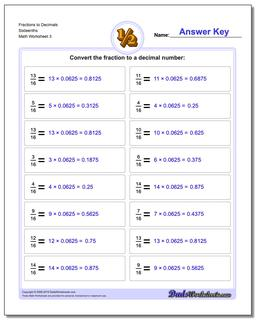# Math Worksheets: Fractions as Decimals: Fractions as Decimals: Fractions to Decimals Sixteenths (Third Worksheet)## Fractions to Decimals Sixteenths (Third Worksheet)

PropertyValue
DescriptionFractions to Decimals Sixteenths: Drills for converting common fractions into decimal equivalents. (Third Worksheet)
Resource TypeWorksheet